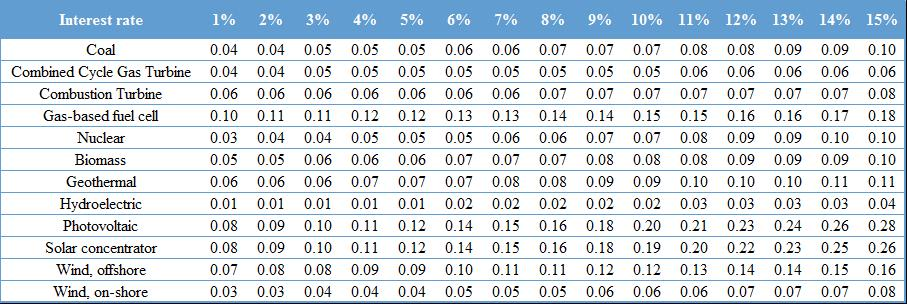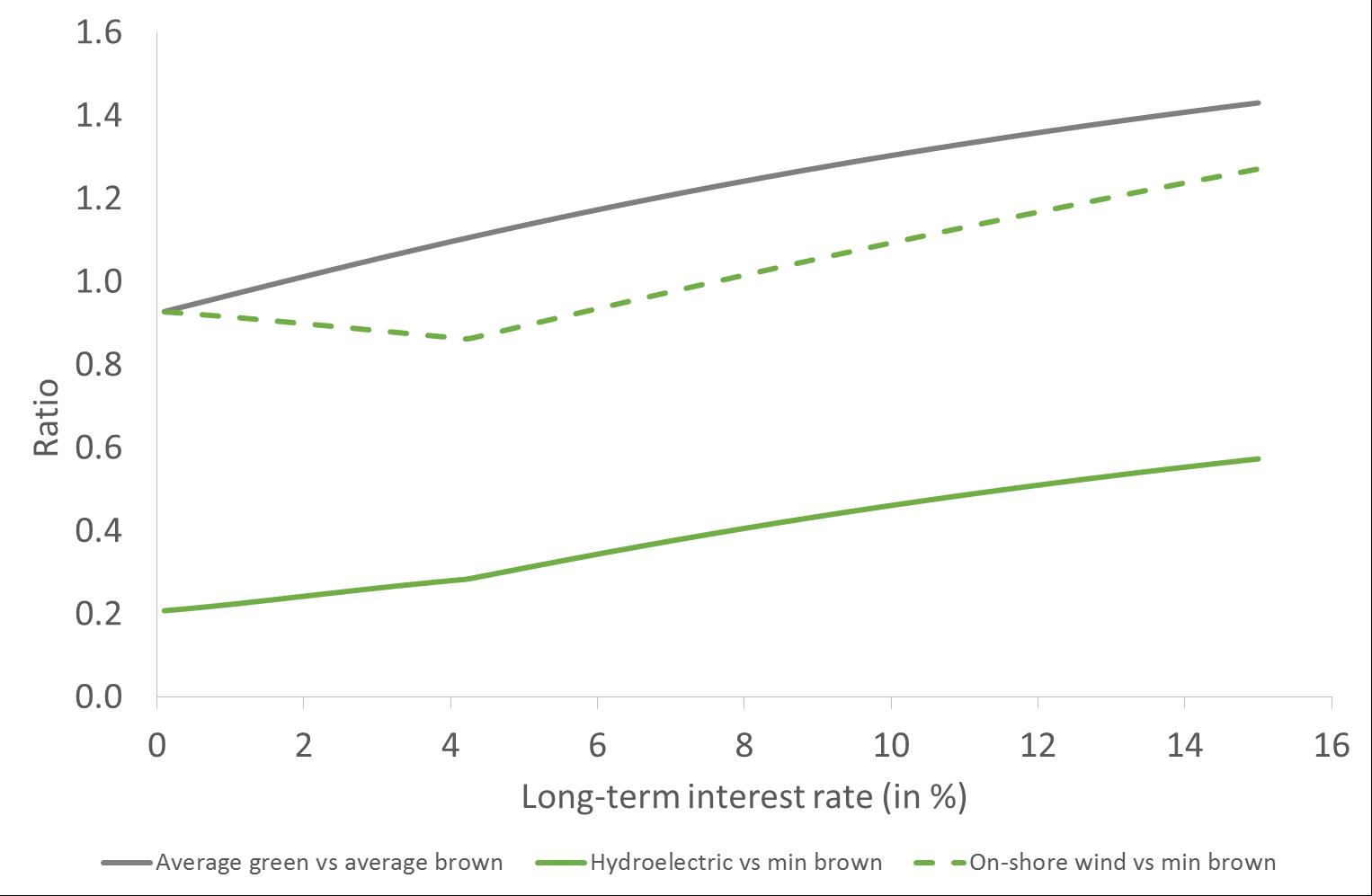# Low Interest Rates: An Opportunity for Renewables

Introduction

Putting the economy on an environmentally sustainable path requires a serious shift into green technologies. Such a shift includes substantial investments in technologies that are based on renewable energy to produce electricity – referred to hereinafter as “green energy technologies”. Interest rates directly and significantly affect the cost of capital for energy projects and are thus a key factor in the decision to invest in such technologies.

Since 2008, partly as a consequence of the quantitative easing policies implemented by central banks, interest rates have decreased considerably and are currently at historic lows. This is potentially good news for green energy technologies, as their capital costs have never been so low. However, this is also true for the capital costs of “brown energy technologies”– i.e. technologies using non-renewable energy sources. Whether the current environment of low interest rates is conducive for an energy transition toward renewables, or not, thus depends on the different magnitude of its effect on the costs of green and brown energy technologies.

In a recent CEP discussion note (Monnin, 2015), we provide an estimation of the relative competitiveness of green versus brown energy technologies for different interest rate levels, by estimating electricity production costs for several technologies. Our results indicate that green energy technologies are more competitive when interest rates are low. They also point out that, in a low interest rate environment, the costs of green energy technologies react stronger to interest rate changes. This suggests that the benefits of low interest rates for green energy technologies’ competitiveness might be partially offset by the risk associated with higher cost volatility.

Methodology and Results

To assess the competitiveness of green energy technologies, we study the impact of interest rates on the levelized cost of energy (LCOE), which is the price at which electricity must be sold for a plant to break even. The data we used to estimate the LCOE of different green and brown energy technologies are taken from the “Transparent Cost Database” collected by Open Energy Information (2014), a U.S. website sponsored by the U.S. Department of Energy, the National Renewable Energy Laboratory and the Renewable Energy and Energy Efficiency Partnership (REEEP). It is important to note that this database collects estimated costs for the United States based on models taken from different studies that have been published between 2008 and 2014, and not observed costs. Our results are thus based on ex ante estimations of the production costs and not on ex post observed costs.

The LCOE of a power plant can be decomposed into three parts: capital costs (the costs of reimbursing the initial investment to build the plant), operation and maintenance costs (the non-fuel costs of running and maintaining the plant) and fuel costs (the costs of fuel to produce power). Interest rates affect capital costs. Table 1 provides the estimated LCOE for green and brown energy technologies at different interest rate levels.

Table 1 – Estimated LCOE for different levels of interest rates (in USD/kWh)Figure 1 shows the relative LCOE for green compared to brown energy technologies, and highlights that green becomes more competitive in a low interest rate environment: At interest rate levels above 2% the average costs of producing electricity are higher for green versus brown energy technologies. With interest rates at 2% the cost ratio of green to brown is 1.0 (i.e. reflecting cost parity) and drops further as interest rates go lower.

Moreover, energy choices are not determined by averages, but rather by the costs of individual technologies. Against this background, Figure 1 also compares the first and second least expensive green energy technologies (hydroelectric and on-shore wind) with the least expensive brown technology (nuclear for interest rates up to 4% and combined cycle gas turbines for higher interest rates). For both green energy technologies, the LCOE ratio is lower for low interest rates, implying that these technologies are more competitive in a low interest rate environment. Note also that the ratio for hydroelectric is below 1 for all interest rate levels, meaning that this technology is always less expensive than any other brown energy technology. On-shore wind is less costly than the least-cost brown technology for interest rates up to 7%.

Figure 1 – Relative LCOE of green energy technologiesIn addition, our results show that on average, the LCOEs for green energy technologies have a higher interest rate elasticity than those for brown energy technologies, especially for low levels of interest rates. The first implication of this is that changes in interest rates will have a stronger impact on green than on brown energy costs. As a result, a decrease in interest rates will translate into a stronger decrease in costs for green compared to brown energy technologies – and may thus potentially stimulate more investment in the former than in the latter.

At the same time, the higher interest rate elasticity of green energy technologies induces greater volatility of their costs in response to interest rate changes. If green energy firms are not completely hedged against interest rate risk, this higher volatility increases cost uncertainty which may translate into a higher risk premium for green energy investments and reduced investments into the sector. This effect is particularly relevant in the current context since the impact of interest rate changes on green energy technologies is greater for low interest rate levels than for high interest rate levels. This suggests that the interest rate risk premium of green energy investments is larger than for brown energy in a low interest rate environment than when interest rates are high.

Conclusions

Our analysis of the impact of interest rates on green energy technologies costs points to three key conclusions:

1) A low interest rate environment makes green energy technologies more competitive. Hydroelectric power is less expensive than any other technology across the entire spectrum of interest rate levels that we analyzed in this paper. Wind on-shore is competitive with all other technologies up until interest rates of 7%.

2) Green energy technologies’ LCOEs react more significantly to a change in interest rates than the LCOEs of brown energy technologies, implying a greater volatility for green energy technologies’ costs in response to interest rate volatility. A higher volatility is damaging because it brings more uncertainty in investment decisions. As a result, stable interest rates are more beneficial for green energy investments than for brown energy investments.

3) In order to increase the ratio of green to brown energy investments, we can consider a discount on the interest rates for the former or a premium on interest rates for the latter. Our estimations indicate that, because interest rate changes have a greater impact on the costs of green energy technologies, a discount on green investments’ interest rates would be more efficient than a premium on brown investments’ interest rates.

References

Monnin, Pierre (2015). “The Impact of Interest Rates on Electricity Production Costs”, CEP Discussion Note 2015/3.

Open Energy Information (2014) “Transparent Cost Database.” Accessed December 12, 2014 at http://en.openei.org/wiki/Transparent_Cost_Database.

 In this note, the term “interest rates” refers to long-term interest rates as short-term interest rates only play a marginal role because of the very long investment horizon required by large electricity-generating plants.

 In May 2015, long-term interest rates were at 0.4% in Japan, at 1.3% in the Euro area, at 2.0% in the United Kingdom and at 2.2% in the United States according to the OECD’s Monthly Monetary and Financial Statistics.